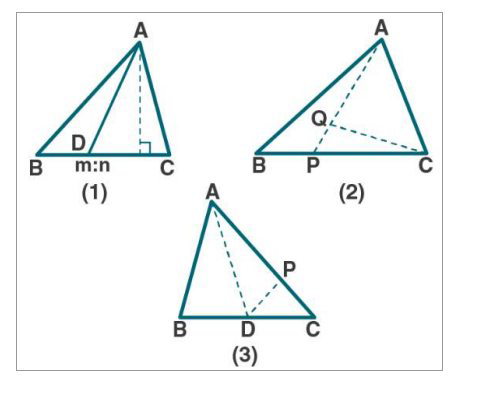# ML Aggarwal Solutions Class 9 Mathematics Solutions for Theorems on Area Exercise 14 in Chapter 14 - Theorems on Area

. (a) In the figure (1) given below, the point D divides the side BC of ∆ABC in the

ratio m: n. Prove that area of ∆ ABD: area of ∆ ADC = m: n.

(b) In the figure (2) given below, P is a point on the side BC of ∆ABC such that PC =

2BP, and Q is a point on AP such that QA = 5 PQ, find area of ∆AQC: area of

∆ABC.

(c) In figure (3) given below, AD is a median of ∆ABC and P is a point in AC

such that area of ∆ADP: area of ∆ABD = 2:3. Find

(i) AP: PC

(ii) area of ∆PDC: area of ∆ABC.(a) Given:

From fig (1)

In ∆ABC, the point D divides the side BC in the ratio m: n.

BD: DC = m: n

To prove:

area of ∆ ABD: area of ∆ ADC = m: n

Proof:

area of ∆ ABD = ½ × base × height

ar (∆ ABD) = ½ × BD × AE ….. (1)

ar (∆ ACD) = ½ × DC × AE ….. (2)

let us divide (1) by (2)

[ar (∆ ABD) = ½ × BD × AE] / [ar (∆ ACD) = ½ × DC × AE]

[ar (∆ ABD)] / [ar (∆ ACD)] = BD/DC

= m/n [it is given that, BD: DC = m: n]

Hence proved.

(b) Given:

From fig (2)

In ∆ABC, P is a point on the side BC such that PC = 2BP, and Q is a point on AP such

that QA = 5 PQ.

To Find:

area of ∆AQC: area of ∆ABC

Now,

It is given that: PC = 2BP

PC/2 = BP

We know that, BC = BP + PC

Now substitute the values, we get

BC = BP + PC

= PC/2 + PC

= (PC + 2PC)/2

= 3PC/2

2BC/3 = PC

ar (∆APC) = 2/3 ar (∆ABC) …… (1)

It is given that, QA = 5PQ

QA/5 = PQ

We know that, QA= QA + PQ

So, QA = 5/6 AP

ar (∆AQC) = 5/6 ar (∆APC)

= 5/6 (2/3 ar (∆ABC)) [From (1)]

ar (∆AQC) = 5/9 ar (∆ABC)

ar (∆AQC)/ ar (∆AQC) = 5/9

Hence proved.

(c) Given:

From fig (3)

AD is a median of ∆ABC and P is a point in AC such that area of ∆ADP: area of ∆ABD

= 2:3

To Find:

(i) AP: PC

(ii) area of ∆PDC: area of ∆ABC

Now,

(i) we know that AD is the median of ∆ABC

ar (∆ABD) = ar (∆ADC) = ½ ar (∆ABC) ……. (1)

It is given that,

ar (∆ADP): ar (∆ABD) = 2: 3

AP: AC = 2: 3

AP/AC = 2/3

AP = 2/3 AC

Now,

PC = AC – AP

= AC – 2/3 AC

= (3AC-2AC)/3

= AC/3 …….. (2)

So,

AP/PC = (2/3 AC) / (AC/3)

= 2/1

AP: PC = 2:1

(ii) we know that from (2)

PC = AC/3

PC/AC = 1/3

So,

= 1/3

ar (∆PDC)/1/2 ar (∆ABC) = 1/3

ar (∆PDC)/ar (∆ABC) = 1/3 × ½

= 1/6

ar (∆PDC): ar (∆ABC) = 1: 6

Hence proved.

Related Questions
Exercises

Lido

Courses

Teachers

Book a Demo with us

Syllabus

Maths
CBSE
Maths
ICSE
Science
CBSE

Science
ICSE
English
CBSE
English
ICSE
Coding

Terms & Policies

Selina Question Bank

Maths
Physics
Biology

Allied Question Bank

Chemistry
Connect with us on social media!